## PICO-8

This guide helps you find the code or commands you need, based on what you want to do.

If you already know the code, and want to search by that, try these:

`PICO-8 Wiki Reference`

`Cheatsheet by Neko250`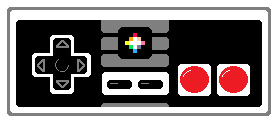• ## PICO-8 Basics

• ### Main ScreenThis is what you will see every time you start up PICO-8. It is a fun retro style command line like the original home computers. You have to type in commands to navigate your folders and load your games.

To begin editing a blank game, just press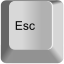• ### Game Editors• ### Code Editor• ### Sprite Editor• ### Map Editor• ### Sound FX Editor• ### Music Editor• ## PICO-8 Commands

• ### Get Help

`help`

lists all commands to navigate PICO-8

• ### See Files and Folders

`dir` or `ls`

dir = "directory" (folder)

ls = "list"

shows all files and folders inside the folder currently open

• ### Open Folder

`cd folder_name`

cd = "change directory" or "change folder"

Opens the folder with that name if it is inside the folder already open

• ### Make Folder

`mk dir folder_name`

mk = "make"

dir = "directory" (folder)

creates a new folder inside the folder already open

• ### Back 1 Folder

`cd ..`

cd = "change directory" or "change folder"

.. = "back"

steps back to the parent folder that holds the folder currently open

`splore`

splore = "explore"

• ### Load Game

`load game_name`

loads the game if that game file is in the folder already open

• ### Save Game

`save game_name`

saves the loaded game into the folder already open

or anywhere in PICO-8, press: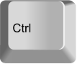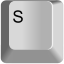• ### Play Game

`run`

Type this into the command screen.

run = play the loaded game

or anywhere in PICO-8, press: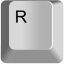• ### Exit Gameexits the running game

• ### Continue Game

`resume`

Resume = "continue"

resumes the running game from last exit point

• ### Edit Game

From PICO-8 command menu, press:Opens game editor of the loaded game

If game is running, press ESC twice

• ### Open Folder on Computer

`folder`

Opens folder on your computer, outside of PICO-8

View the actual saved PICO-8 files and folders.

• ### Set Player Buttons

`keyconfig`

"keyconfig" = key configuration (button set up)

Opens button editor

• ### Restart PICO-8

`reboot`

Reboot = "restart"

restarts the whole PICO-8 system, make sure you save first!

• ### Exit PICO-8

`shutdown`

closes the whole PICO-8 system, make sure you save first!

• ## Getting Started Coding

• ### Set up the Game

``function _init()``

init = "initiate"

do something one time when game starts

not needed for the game to run

• ### Update the Game

``function _update()``

do something every frame we see: 30 times per second

Frame: 1 picture on screen.

Needed for game to run.

``function _update60()``

or use this for 60 times per second

• ### Draw to the Game Screen

``function _draw()``

do something every frame we see: 30 times per second

Frame: 1 picture on screen

Needed for game to run

• ## Screen

• ### Write on Screen

`` print(words, [x, y], [color])``

print = write words on the screen, at [x, y] place, in color

x = number of pixels from the top left corner going right

y = number of pixels from the top left corner going down

[x,y] is not needed, [color] is not needed

• ### Set Position on Screen

`` cursor(x, y)``

cursor = imagine a mouse pointer on the screen(PICO-8 games do not have a mouse)

x = number of pixels from the top left corner going right

y = number of pixels from the top left corner going down

• ### Erase Screen

`` cls()``

CLS = "clear screen"

erases the entire screen

Erasing and re-drawing everything on the screen every frame is standard practice. So you will almost always want to have this in the first line of the `_draw()` function.

• ### Move camera

`` camera(x, y)``

sets the camera to position on screen

x = number of pixels from the top left corner going right

y = number of pixels from the top left corner going down

`` camera()``

reset camera to 0,0 position

• ### Cut out sides of screen

`` clip(x, y, w, h)``

Clipping is when you cut out the sides, like cropping a picture

x = number of pixels from the top left corner going right

y = number of pixels from the top left corner going down

w = width of clipping rectangle in pixels

h = height of clipping rectangle in pixels

``clip()``

Reset to fullscreen, no clipping

• ## Draw Shapes

• ### Circle Outline

`` circ(x, y, radius, [color])``

circ = circle

x = number of pixels from the top left corner going right

y = number of pixels from the top left corner going down

x,y = the position of the center of the circle

radius = number of pixels from the center to the outside

[color] = color number (optional)

• ### Circle Filled

`` circfill(x, y, radius, [color])``

circfill = circle filled

x = number of pixels from the top left corner going right

y = number of pixels from the top left corner going down

x,y = the position of the center of the circle

radius = number of pixels from the center to the outside

[color] = color number (optional)

• ### Line

`` line(x1, y1, x2, y2, [color])``

circ = circle

x = number of pixels from the top left corner going right

y = number of pixels from the top left corner going down

x1, y1 = the position of the start of the line

x1, y1 = the position of the end of the line

[color] = color number (optional)

• ### Rectangle Outline

`` rect(x1, y1, x2, y2, [color])``

rect = rectangle

x = number of pixels from the top left corner going right

y = number of pixels from the top left corner going down

x1, y1 = the position of the first corner

x1, y1 = the position of the opposite corner

[color] = color number (optional)

• ### Rectangle Filled

`` rectfill(x1, y1, x2, y2, [color])``

rectfill = rectangle filled

x = number of pixels from the top left corner going right

y = number of pixels from the top left corner going down

x1, y1 = the position of the first corner

x1, y1 = the position of the opposite corner

[color] = color number (optional)

• ## Colors

• ### Color Numbers

`00` (#000000) black
`01` (#1D2B53) dark blue
`02` (#7E2553) dark purple
`03` (#008751) dark green
`04` (#AB5236) brown
`05` (#5F574F) dark_gray
`06` (#C2C3C7) light_gray
`07` (#FFF1E8) white
`08` (#FF004D) red
`09` (#FFA300) orange
`10` (#FFFF27) yellow
`11` (#00E756) green
`12` (#29ADFF) blue
`13` (#83769C) indigo
`14` (#FF77A8) pink
`15` (#FFCCAA) peach• ### Select Color

`` color( color number )``

select the color to be used

This will set the color to be used in the next use, such as: `print() circ() rect() line()`

If those and functions like them do not use the optional color setting, then whatever color was last set will be used. By using the optional color settings in functions that have them, it sets the new color to be the currently selected color.

Example:

``````color(8)    --#8 is red
print("hello")
--letters will be red``````

The above is the same as:

``````print("hello",0,0,8)    --0 for x, 0 for y, 8 for red
--letters will be red``````

This is how they work together:

``````print("hello",0,0,8)    --0 for x, 0 for y, 8 for red
color(7)  --#7 is white
print("world")
--hello will be red
--world will be white``````

• ### Get Color from Screen

`` pget( x, y )``

`pget` = "pixel get"(1 pixel)

x = number of pixels from the top left corner going right

y = number of pixels from the top left corner going down

Returns the color number of the pixel at location (x,y) on the screen.

• ### Set Color to Screen

`` pset( x, y, [color] )``

`pset` = "pixel set"(1 pixel)

x = number of pixels from the top left corner going right

y = number of pixels from the top left corner going down

[color] = 0-15 color number, not needed

• ### Get Color from Sprite Sheet

`` sget( x, y )``

`sget` = "sprite sheet get"(1 pixel)

x = number of pixels from the top left corner going right

y = number of pixels from the top left corner going down

Returns the color number of the pixel at location (x,y) on the sprite sheet (not screen).

Both x and y must be within the range of 0 and 127.

• ### Set Color to Sprite Sheet

`` sset( x, y, [color] )``

`sset` = "sprite set"(1 pixel)

x = number of pixels from the top left corner going right

y = number of pixels from the top left corner going down

[color] = 0-15 color number, not needed

• ### Replace Colors

`` pal( color_from, color_to, [palette])``

`pal` = "palette"(set of colors)

color_from = color number to change

color_to = color number to change to

[palette] = 0 for next draw or 1 for already drawn (optional, default 0)

`` pal()``

resets all colors to the default palette

• ### Make Color Transparent

`` palt( color, transparent )``

`palt` = "palette transparent"

color = color number

transparent = true or false

true = do not draw this color pixel

false = do draw this color pixel

`` palt()``

resets all colors to default transparency, black (0) is transparent

• ## Sprites

• ### What is a Sprite?

sprite = a drawing using multiple pixels that can be considered as a single thing.

A character, item, or piece of the background are usually made of one or many sprites. Multiple sprites that look similar but have small changes between them are often used to create sprite animations.

A sprite has a width (W) and a height (H). The W is the number of pixels wide and the H is the number of pixels tall.
We can communicate the size of a sprite by saying these two numbers together.
The size of a sprite is called its "dimensions".As you can see, the bigger sprite looks better because it has more pixels and can show more detail. In fact, even the most beautiful and detailed digital pictures are just made of many many pixels. Here is how we can take the same character and draw him in larger dimensions in PICO-8.• ### What is a Sprite Sheet?

sprite sheet = a collection of multiple sprites.

You can find the PICO-8 sprite sheet in the sprite editor. Each PICO-8 game has one sprite sheet that appears broken up into 4 pages. The sprite editor is more than just a place to draw our sprites:Example of a full sprite sheet (1st page):Here, you can see multiple sprites in a sprite sheet used to create movement animation.
Each small monkey is a single tile (8x8) and there are 9 different sprites that are used to make it look like one monkey is running in the game.Hover your mouse over a selected sprite's top left corner and you will see the x and y values of the sprite location on the sprite sheet. This is useful for the SSPR( ) function.

• ### Draw a Sprite on Screen

``spr(sprite #, x, y, [w], [h], [flipx], [flipy])``

#### SPR( ) Full Tutorial!

spr = "sprite"

sprite # = a number of the sprite in the sprite sheet

x = a number of pixels from the top left corner of the game screen going right

y = a number of pixels from the top left corner of the game screen going down

w = a number for how wide the sprite is in tiles (8 pixels in 1 tile) Default = 1

h = a number how tall the sprite is in tiles (8 pixels in 1 tile) Default = 1

flipx = true or false for flipping or reversing the sprite from left to right / right to left

flipy = true or false for flipping or reversing the sprite from top to bottom / bottom to top

If you do not include columns and rows, the default is 1. So the simplest form of this code draws an 8x8 sprite.

Example of a 16x16 pixel sprite:A sprite is always drawn starting from the top left corner of the sprite. So the X and Y coordinates given will be the location for the top left pixel of the sprite.

If you flip the sprite on the X or Y, this only changes the way it is drawn, not the position on the screen.

• ### Draw a Sprite of Any Size

``sspr( sx, sy, w, h, x, y, [dw, dh], [flipx], [flipy] )``

`sspr` = "special sprite" ?

sx = "sprite x" number of pixels right in sprite sheet to the top left corner of the sprite

sy = "sprite y" number of pixels down in sprite sheet to the top left corner of the sprite

w = "width" number of pixels wide from sx

h = "height" number of pixels high from sy

x = # pixels from the left to start drawing sprite on screen

y = # pixels from the top to start drawing sprite on screen

dw = the width of the sprite drawn on the screen. Default = w

dh = the height of the sprite drawn on the screen. Default = h

flipx = If true, the image is drawn inverted left to right. Default = false

flipy = If true, the image is drawn inverted top to bottom. Default = false

A sprite is always drawn starting from the top left corner of the sprite. So the X and Y coordinates given will be the location for the top left pixel of the sprite.

If you flip the sprite on the X or Y, this only changes the way it is drawn, not the position on the screen.

• ## Maps

• ### Draw Part of Map on Screen

``map( tile x, tile y, x, y, w, h, [layer] )``

tile x = column number of top left corner of map section in tiles (8 pixels per tile)

tile y = row number of top left corner of map section in tiles (8 pixels per tile)

x = # pixels from the left to start drawing map section on screen

y = # pixels from the top to start drawing map section on screen

layer = use only the sprites with this flag number.

• ### Get Sprite on Map

``mget( column, row )``

`mget` = "map get"

columns = number of tiles from the top (8 pixels in 1 tile)

rows = number of tiles from the right (8 pixels in 1 tile)

Example:
``````playerColumn = playerX / 8
playerRow = playerY / 8
mget( playerColumn + 1, playerRow )``````

This is useful for finding certian things on the map near the player. In the code above, you get what sprite number is to the right of the player on the map. For example, I might want to check for the walls on the map.

• ### Set Sprite on Map

``mset( column, row, sprite number )``

`mset` = "map set"

columns = number of tiles from the top (8 pixels in 1 tile)

rows = number of tiles from the right (8 pixels in 1 tile)

sprite number = number of the sprite in the sprite sheet

Example:
``````playerColumn = playerX / 8
playerRow = playerY / 8
doorColumn = 4
doorRow = 5
doorOpen = 20 --sprite #
doorClose = 21 --sprite #
if playercolumn == doorcolumn and playerrow == doorrow then
mset( doorcolumn, doorrow, dooropen )
else
mset( doorcolumn, doorrow, doorclose )
end``````

This is useful for changing certain things on the map, when something happens. In the code above, we check if the player is standing on a door, then set the door sprite to be open, else set it closed.

• ## Strings and Variables

• ### What is a String?

``"any text inside quotation marks"``

A string is a line of text. It can be one letter or a full sentence, or just symbols.

This tells the computer to see anything inside quotation marks (" ") as just letters and numbers, not variables.

Example:
``````a = hello
print( a ) --prints [nil]``````

nil (null, nothing) printed because the computer was looking for a variable called HELLO, not a string.

``````B = "hello"
print( B ) --prints: hello``````

• ### Count the Length of the String

``#(string)``

Placing a # in front of a string counts how many characters are in the string.

Example:
``````a = "hello"
print( #a ) -- prints: 5``````

• ### What is a Variable?

``(variable) =(information)``

A variable is a letter or word that holds information.

This tells the computer to make the variable hold the information and remember them.

Example 1:
``````a = 1
print( a ) --prints: 1``````

Anytime the Computer sees A, it will remember that A is 1.

Example 2:
``````b = 20
c = 10
print( b + c ) --prints: 30``````

The computer understands B as 20 and C as 10 so adding B and C, the computer adds 20 and 10 and prints the answer.

• ### Mix Strings with Variables

``(string)..(variable)``

the .. tells the computer to read a string and then change to read a variable.

Example:
``````score = 10
print( "score= "..points ) --prints: score= 10``````

• ## Tables

• ### What is a table?

Table = a set of information placed into rows and columns

You can collect and organize your game information easily into tables.

You can't see the tables in PICO-8, so you will have to imagine them.

Example:
 Column 1(key) Column 2(value) 1 "A" 2 "B" 3 "C"

Key = The first column's data. A number or string used to find the data stored in Column 2

Value = The second column's data. A number, string, boolean, or table that you want to store

• ### Create an Empty Table

``table_name={ }``

{ = starts the table

} = ends the table

Example:
``fruit={ }``
 Fruit

• ### Create a Table as a Simple List

``table_name={ a, b, c }``

{ = starts the table

} = ends the table

a, b, c, = strings or variables added to the table and put in rows 1, 2, and 3 automatically

Example:
``colors={ "red", "blue", "pink", "yellow" }``
 Colors 1 "red" 2 "blue" 3 "pink" 4 "yellow"

• ### Create a Table with Named Keys

``table_name={ a=b, c=d, e=f }``

{ = starts the table

} = ends the table

a=b : information added to the table and put in key: "A" linked with value: "B"

Example:
``highscores={ john=10, bob=143, kim=984 }``

Or write it like this:

``````highscores={}      --creates empty table
highscores.john=10 -- adds key "john" with value set to 10
highscores.bob=143
highscores.kim=984``````

This is what it would look like:

 HighScores John 10 Bob 143 Kim 984

• ### Create a Table inside another Table

``table_name={ a={ }, b={ } }``

{ = starts the table

} = ends the table

A{ } : First table(named A) inside main table

B{ } : Second table(named B) inside main table

Example:
``````enemies={
{health=10,attack=3},
{health=20,attack=8}
}``````
Enemies
1  Health 10 Attack 3
2  Health 20 Attack 8

• ### Add to a Table

``add( table, value )``

table = the variable name of the table

value = the information to add to the table

, = commas separates the table name with the information to add

Example:
``colors={ "red", "blue", "pink", "yellow" }``
 Colors 1 "red" 2 "blue" 3 "pink" 4 "yellow"
``add( colors, "purple" )``
 Colors 1 "red" 2 "blue" 3 "pink" 4 "yellow" 5 "purple"

• ### Delete from a Table

``del( table, value )``

table = the variable name of the table

value = the information to delete from the table

, = commas separates the table name with the information to delete

Example:
 Colors 1 "red" 2 "blue" 3 "pink" 4 "yellow"
``del( colors, "blue" )``
 colors 1 "red" 2 "pink" 3 "yellow"

Notice that BLUE was key #2. Deleting BLUE, also moved the rest of the list up one number.

This happens for tables that have keys as numbers, but it does not change tables that have keys as strings.

• ### Get the Total Number of things in a Table

``#table``

# = count the number of items in a table

Example:
 HighScores john 10 bob 143 kim 984

``#highscores --count: 3``

• ### Set Value in a Table

``table[key]=value``

[ ] = the key of the table(left column)

Example:
 HighScores john 10 bob 143

``highscores["john"]=30``
 HighScores john 30 bob 143

Or:

``table[number]=value``

[ ] = the key of the table(left column)

Example:
 Colors 1 ""red" 2 "blue" 3 "pink"

``colors="orange"``
 colors 1 "red" 2 "orange" 3 "pink"

• ### Get Value from a Table with Numbered Keys

``table[number]``

[#] = the number key of the table (left column)

Example:
 Colors 1 "red" 2 "orange" 3 "pink"

``print( colors ) --prints: orange``

• ### Get Value from a Table with Named Keys

``table[key] <-or-> table.key``

[ ] = the variable name key of the table (left column)

This is just like a variable, so just asking for the table and key, it will be whatever the value is in the table at that key.

Example:
 HighScores john 10 bob 143

``print( highscores["john"] ) -- prints: 10``
``print( highscores.john ) -- prints: 10``

• ## Loops

• ### Repeat this a Certain Number of Times

``````for variable = start, stop, count_by do
--code to repeat
END``````

variable = a letter or word to set as the current repeat count

start = a number for the repeat count to start at

stop = a number for the repeat count to end at

count by = a number for the count to increase or decrease by (optional, default 1)

"FOR LOOPS" begin counting from the "start" number, count up or down by the "count by" number, and stops at the "end" number.

To count up, make the "count by" number positive.

To count down, make the "count by" number negative.

Example:
``````for i = 1, 9, 2 do
print(i)
end

--prints: 1 3 5 7 9``````

If the "count by" number is left out, then it will automatically be 1.

Example:
``````for i = 1, 9 do
print(i)
end

--prints: 1 2 3 4 5 6 7 8 9``````

If you want to start at a high number and count down, then make the "count by" number a negative number.

Example:
``````for i = 10, 3, -1 do
print(i)
end

--prints: 10 9 8 7 6 5 4 3``````

• ### Repeat this Until something Happens

``repeat (code) until (variable is true)``

code = whatever code you want to repeat

variable = a letter or word that is keeping track of something

"REPEAT LOOPS" continue doing the code after REPEAT and stops repeating when the UNTIL variable is true.

Example:
``````x=0
repeat
print(x)
x+=1
until x==5

--prints: 0 1 2 3 4``````

• ### Repeat this While something is Happening

``````while (variable) do
(code)
end``````

variable = a letter or word that is keeping track of something

code = whatever code you want to repeat

"WHILE LOOPS" repeat the code after DO and stops repeating when the variable is true.

Example:
``````x=4
while x<10 do
print(x)
x+=1
end

--prints: 4 5 6 7 8 9``````

• ### Repeat this For Each Item in a Table

``foreach( table, function )``

table = name of table

function = name function to do

Example:
 HighScores john 10 bob 143 kim 984

``foreach(highscores, sort)``

From the first to the last item in HIGHSCORES table, put it through the SORT function.

FOREACH gives the table value to the function so SORT function should be `sort(value)`.

• ### Repeat this for All Items in a Table

``for value in all(table) do``

value = any variable name to set as the table item in the right column

table = name of table

Example:
 HighScores john 10 bob 143 kim 984
``````for score in all(highscores)
print(score)
end

-- prints: 10 143 984``````
``for key, value in pairs(table) do``

key = any variable name to set as the table item in the left column

value = any variable name to set as the table item in the right column

table = name of table

``````for name, score in pairs(highscores)
print(name)
print(score)
end

-- prints: John 10 Bob 143 Kim 984``````

• ## Save and Export

• ### Save Game

`save gamename`

Type this into the command screen of PICO-8.

Or, anywhere in PICO-8, do this:• ### Save Game Label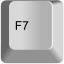This takes a screen shot of your game, and saves it as the game's label when creating the game cartridge.

• ### Save Game Cartridge

`save gamename.p8.png`

Type this into the command screen of PICO-8.

This saves your game cartridge as an image, with the label on the cover.

You can share this image with others who have PICO-8 and they can play it in PICO-8.

WAIT! Before you save your game image, you should write this in the first two lines of your game's code and it will show on your game cartridge:

``````--(game name)

Example:• ### Export Game as Website

`export gamename.html`

Type this into the command screen of PICO-8.

This exports the game for websites. It gives you two files that need to stay together. One is .html and the other is .js

• ### Export Game as Desktop App

`export gamename.bin`

Type this into the command screen of PICO-8.

This exports the game as a standalone application. Share your game with anyone! They don't need PICO-8 to play it.

• ### Export Sprite Sheet

`export gamename.png`

Type this into the command screen of PICO-8.

This exports the sprite sheet as a picture.

• ### Export Sound FX

`export gamename_sfx.wav`

Type this into the command screen of PICO-8.

This exports the game as a standalone application. Share your game with anyone! They don't need PICO-8 to play it.

• ## Controls (Inputs)

• ### Check if Button is Pressed

``btn( # , [player #] )``

If you hold the button down, this will act like it is rapidly pressed.

``btnp( # , [player #] )``

If you hold the button down, this limits one press every other game frame.For multiplayer games, you will need to include player numbers: 0-7

Instead of button numbers, you can also use the symbols.

 Button Number Symbol Left 0 shift+L Right 1 shift+R Up 2 shift+U Down 3 shift+D O 4 shift+O X 5 shift+X

• ## Music and Sound Effects (SFX)

• ### Play a short Sound Effect

``sfx( sound-number, [channel], [offset] )``

sound-number = the number in the sound editor.

channel = which of the 4 channels {0, 1, 2, 3} (optional)

offset = when (in the sound) to start playing the sound. (# of notes, optional)

If sound is -1, current sound on the channel is stopped

If sound is -2, it will finish the sound but stop looping.

If channel is -1 (default), a channel that is not in use will be picked automatically.

Example:

``sfx(1) -- play sound FX number 1``
``sfx(3, 0) -- play sound FX 3 in channel 0``
``sfx(4, 3, 6) -- play sound FX 4 in channel 3, start 6 notes in``

• ### Play Music

``music( music-number, [channel], [offset] )``

music-number = the number of the song in the music editor.

channel = which of the 4 channels to play in {0, 1, 2, 3} (optional)

offset = when (in sound FX) to start playing the sound. (# of notes, optional)

Example:

``music(1) -- play music number 1``
``music(3, 0) -- play music 3 in channel 0``
``music(4, 3, 8) -- music 4, channel 3, start 8 notes in``

• ## Math

• ### Round a number down

``flr( # )``

`flr` = "floor"(nearest whole number down)

# = any number

Example:

``a = flr( 1.6 ) -- a = 1``
``b = flr( 7 / 3 ) -- b = 2``

• ### Make a number Positive

``abs( # )``

`abs` = "absolute" same number but ignore the sign( + / - )

# = any number

Example:

``a = abs( 16 ) -- a = 16``
``b = abs( -2.5 ) -- b = 2.5``
``d = abs( 1.5 - 2 ) -- d = 0.5``

• ### Get a number's sign: ( + / - )

``sgn( # )``

`sgn` = "sign" positive or negative ( + / - )

# = any number

If # is positive, SGN(#) = 1

If # is zero, SGN(#) = 1

If # is negative, SGN(#) = -1

Example:

``a = sgn( 16 ) -- a = 1``
``b = sgn( 0 ) -- b = 1``
``c = sgn( -2.5 ) -- c = -1``
``d = sgn( 5 - 16 ) -- d = -1``

Notice that SGN( 0 ) returns 1, simply as a non-negative number.

This is a handy function for recognizing 0 and returning: ( 1 / 0 / -1 )

``````function truesgn(a)
local r=0
if (a!=0) r=sgn(a)
return r
end``````

(shared by dw817)

• ### Square Root

``sqrt( # )``

`sqrt` = "square root"

# = any number

What is Square Root?

To square a number, means you times the number by itself:
3 x 3 = 9 or "3-squared" = 9

Square Root is the opposite and finds what number times itself equals the #:
"square-root of 9" = 3

Example:

``a = sqrt( 16 ) -- a = 4``
``b = sqrt( 100 ) -- b = 10``
``c = sqrt( 30 - 21 ) -- c = 3 ``

• ### Get Larger Number

``max( # , [#] )``

`max` = "maximum"

# = any number

This compares two numbers and gives the higher value

(The second number is optional, defaults to 0)

Example:
``a = max( 16 , 25 ) -- a = 25``
``a = max( 5 ) -- a = 5``
``a = max( -10 ) -- a = 0``
``b = max( 20+30 , 100-30 ) -- b = 70``

• ### Get Smaller Number

``min( # , [#] )``

`min` = "minimum"

# = any number

This compares two numbers and gives the lowest value.

(The second number is optional, defaults to 0)

Example:
``a = min( 16 , 25 ) -- a = 16``
``a = max( 5 ) -- a = 5``
``a = max( -10 ) -- a = 0``
``b = min( 20+30 , 100-30 ) -- b = 50``

• ### Get Middle Number

``mid( # , # , # )``

MID = "middle"

# = any number

This sorts a list of numbers then gives the middle (median) number of the list.

Example:
``a = mid( 25 , 10 , 40 ) -- a = 25``
``b = mid( 20+30 , 100-30 , 10+50 ) -- b = 60``

• ### Make a Wave

``sin( # ) -- sine wave``
``cos( # ) -- cosine wave``
``atan2( # , # ) -- arc tangent``
Examples:
Purple = Cosine
Green = Sine• ### Random Number: Decimal

``rnd( # )``

`rnd` = "random"

# = any number

Generates a random number from 0 to #(including 0 and #)

Example:
``a = rnd( 8 ) --gives a decimal between 0 and 8``
``b = rnd( 20+30 ) --gives a decimal between 0 and 50``

• ### Random Number: Whole #

``flr( rnd( # ) )``

`rnd` = "random"

`flr` = "floor" (round down)

# = any number

Generates a random number from 0 to #(including 0 and #)

Example:
``a = flr( rnd( 3 ) ) --gives whole number between 0 and 3``
``b = rnd( 5*10 ) --gives a decimal between 0 and 50``

This is a menu and coding reference for Pico-8.

`font`